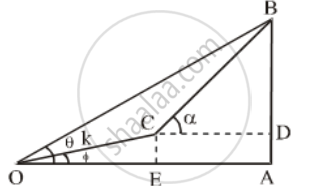Share
Notifications

View all notifications
Books Shortlist
Your shortlist is empty

# The Angle of Elevation of a Cliff from a Fixed Point is θ. After Going up a Distance of K Metres Towards the Top of Cliff at an Angle of φ, It is Found that the Angle of Elevation is α. - CBSE Class 10 - Mathematics

Login
Create free account

Forgot password?

#### Question

The angle of elevation of a cliff from a fixed point is θ. After going up a distance of k metres towards the top of cliff at an angle of φ, it is found that the angle of elevation is α. Show that the height of the cliff is metres

#### Solution

Let AB be the cliff and O be the fixed point such that the angle of elevation of the cliff from O is θ i.e. ∠AOB = θ. Let ∠AOC = φ and OC = k metres. From C draw CD and CE perpendiculars on AB and OA respectively.

Then, ∠DCB = α.

Let h be the height of the cliff AB.In ∆OCE, we have

\sin \phi =\frac{CE}{OC}

\Rightarrow \sin \phi =\frac{CE}{k}

⇒ CE = k sin φ …(i) [∵ CE = AD]

⇒ AD = k sin φ

\text{And, cos}\phi =\frac{OE}{OC}

\Rightarrow \text{cos}\phi =\frac{OE}{k}

⇒ OE = k cos φ ….(ii)

In ∆ OAB, we have

\text{tan }\theta =\frac{AB}{OA}

\Rightarrow \text{tan }\theta =\frac{h}{OA}

⇒ OA = h cot θ ….(iii)

CD = EA = OA – OE

= h cot θ – k cos φ …..(iv) [Using eqs.(ii) and (iii)]

and, BD = AB – AD = AB – CE

= (h – k sin φ) ….(v) [Using equation (i)]

In ∆BCD, we have

\text{tan}\alpha =\frac{BD}{CD}\Rightarrow \text{ tan}\alpha=\frac{h-k\sin \varphi }{h\cot \theta -k\cos \varphi }

[Using equations (iv) and (v)]

\Rightarrow \frac{1}{\cot \alpha }=\frac{h-k\sin \varphi }{h\cot \theta-k\cos \varphi}

⇒ h cot α – k sin φ cot α = h cot θ – k cos φ

⇒ h(cot θ – cot α) = k(cos φ – sin φcot α)

h= k((cos φ – sin φcot α))/((cot θ – cot α))

Is there an error in this question or solution?

#### Video TutorialsVIEW ALL 

Solution The Angle of Elevation of a Cliff from a Fixed Point is θ. After Going up a Distance of K Metres Towards the Top of Cliff at an Angle of φ, It is Found that the Angle of Elevation is α. Concept: Heights and Distances.
S